Next: The format for mcf.bin' Up: The format for mcf.asc' Previous: The format for mcf.asc'   Contents

## Specifics

For the reader who needs to know the exact format and content of the mcf.asc' file, it is as follows.

First line:
The form of the first line is,
 WRITE (iocmcf,
&  '("MCFGEN  Date:", i8, 5x, "Library type:", i3)')
&    i_date, nclibtyp

Here, the date is in the format such that 12 January 1995 is written as 950112. The code for the library type is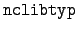= 0
means group-averaged data.= 1
means grid-based data.

Second line:
The library source and the date are given as

WRITE(iocmcf,'("Source Library Id Word:", i12,
&  5x, "Last Changed:", 2x, i6)')
&    libid, libdate

Here, libid is meant to be the library identifier packed in some format, but it is not clear what the components are. The convention given on page 16 of reference  is only followed for incident photons, because all of the other mcf.asc' files have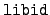= 103940000.

Identify the target:
A line identifying this target, the number of energy groups, and the number of reactions.
    WRITE(iocmcf,'(i6, 12x, i3, 3x, i3,44x,"*")')
& nnza, negi, numcs

Here, the meaning of the entries is:

nnza:
The atomic number and mass number of the target, formatted as 1000*Z + A. There are some special conventions. A value of A = 0 denotes the natural element, so that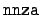= 8000 is natural oxygen. The value= 99120 denotes a short-lived fission fragment, and the value= 999999 is a flag marking the end of the file.

negi:
The number of energy groups.

numcs:
The number of reactions (C-values) for this target.

*:
The final "*" on the line is a flag showing that we are starting data for a new target.

Basic target data:
Identify the incident particle, the halflife, the excitation level of the target, the temperature of the target, the target's atomic mass, and the library date.
    WRITE(iocmcf,'(1p, i2,4e11.4,1x,i6)')
& iyi, halflife, elvfd, tmpfd, atm, rpdate

The meaning of the entries is:

iyi:
The identifier for the incident particle.
halflife:
The halflife of the target. A stable target is given a halflife of 1050.
elvfd:
The excitation level of the target.
tmpfd:
The temperature of the target.
atm:
The atomic weight of the target.
rpdate:
The date of the data for this target.

List the reactions:
Then follows the list of values of the C reaction identifier for this target.
    WRITE(iocmcf, '(24i3)')
& (C_clean(c_count), c_count=1, numcs)


Data block:
A data block takes the following form.
Flag:
A single line, starting with a flag to identify the type of data. The most common format for the data-flag line is,
    WRITE(iocmcf, '(i4, i7, 1x, a8)')
& flag, number, string


There is usually a second line identifying the reaction and the type and number of data entries.

Data:
The actual data.

The type of data is identified by means of the flag. The dentification is unique in all cases except that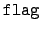= 12 has two interpretations--its most common usage is as the flag for energy distributions (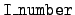= 4), but it also used for the angular distribution of gammas. The header line must be used to distinguish them. A point to be aware of is that for= 1 and 2 there is no header line. But these two data blocks appear only with the first target, and they come immediately after the list of reactions, before the rest of the data. We list the types of data blocks ordered in terms of flag because that is what is used by the code mcfbin which reads the mcf.asc' file. The user who is accustomed to working in terms of the data identifier I_number should consult the following list.= 0:
Cross sections, data with= 4, 5, and 6 are given, in that order. For the first target, however, this data is preceeded by the lists of energy group boundaries (= 1) and target temperatures (= 2).= 1:
Angular distributions,= 10 and sometimes 12. When= 12 data is present, it comes first.= 3:
Correlated energy-angle distributions,= 11.= 4:
Energy distributions,= 12.= 7:
Fission neutron multiplicities,= 3.= 9:
Photon multiplicities,= 9.= 10:
Energy deposition,= 7.= 12:
Average available energy,= 6.= 941:
Coherent scattering form factors,= 14.= 942:
Incoherent scattering form factors,= 15.

The content of the data blocks as ordered by flag is as follows.= 1:
This block of data is energy group boundaries, number is the number of groups, and string is "gp bds". There is no header, and the energy group boundaries follow immediately, written with the format,
WRITE(iocmcf, '(1p, 6e11.4)') (ebound(j), j=1, nebi)= 2:
This block of data is target temperatures, number is the number of temperatures, and string is "temp.". There must be only one temperature because Doppler broadening is not now done in mcfgen. That temperature is given on the next line in the format,

WRITE(iocmcf, '(1p, 6e11.4)')
&  (temture(j), j=1, numtemp)= 3:
This block of data is multiplicity of fission neutrons. The format of the flag line is nonstandard,
     WRITE(iocmcf, '(i4, i2, 1x, a16)')
& flag, number, string

For prompt neutrons we have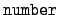= 0 and the label string is "nubar, prompt". For delayed neutrons we have the identifier= 1 and string is "nubar, delayed". The flag line is followed by a header of the form,
      WRITE(iocmcf, '(1p, 5i5, 5x, 3e12.5)')
& c, I_number, s, igsrt, igend, q0, x1

The meaning of these entries is:

c:
The C reaction identifier (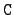= 15 for fission).

I_number:
For= 3 the data identifier I_number is 7.

s:
The S reaction identifier. It determines the meaning of x1.

igsrt:
Identify the first nonzero cross section. (This amounts to an energy threshold.)

igend:
Identify the highest nonzero cross section. (In effect, this is a maximum energy.)

q0:
The energy of the reaction.

x1:
The first extra information, depending on the value of s.

The group-averaged multiplicities are printed in the format,
     WRITE(iocmcf, '(1p, 6e11.4)')
& (array(j), j=igsrt, igend)= 4:
This block of data is flux-weighted group-averaged cross sections, number is the date for the data, and string is "gp x-sec". The flag line is followed by a header of the form,
     WRITE(iocmcf, '(1p, 6i5, 3e12.5)')
& c, I_number, s, igsrt, igend,
& kin_type, q0, x1, tempture

The differences from the header for= 3 are

I_number:
The data identifier is 0.
kin_type:
The kinematics type for this reaction.
tempture:
The temperature of the target.

The cross section data follows,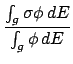and it is written in the following format.
     WRITE(iocmcf, '(1p, 6e11.4)')
& (gpsig0(j), j=igsrt, igend)


The cross section data is followed immediately in the file (without an identification flag) by a line containing the number of particles produced by this reaction, including the residual nucleus.

     WRITE(iocmcf, '(i2)') parts_produced(1, nrc)

Then come the secondary particles and their multiplicities as pairs (yo, multiplicity). The residual nucleus is the last particle, unless it is light enough to be included with the other secondary particles.
     WRITE(iocmcf, '(15i6)') (parts_produced(j, nrc),
&  j=2, parts_produced(1, nrc)*2 + 1)= 5:
This block of data is the calculated energy available, and it always follows the block for= 4. The flag line is nonstandard in that number is not present. The string in the flag line is "en avail". The header line from= 4 is printed again. (We again have= 0.) The available energies per group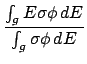are then given with the format,
     WRITE(iocmcf, '(1p, 6e11.4)')
& (av_en_avail(j), j=igsrt, igend)= 6:
If this block of data is present, it appears immediately after the block for= 5. It contains the energy available as given in the Production Cross Section library file. In the flag line number is not present, and string is "Q-bar". The header line from= 4 is printed again. (We again have= 0, but now= 5.) The available energies per group are then given with the format,
     WRITE(iocmcf, '(1p, 6e11.4)')
& (qbargp(j), j=igsrt, igend)= 7:
This block of data is energy carried by a secondary particle, number identifies the first of the list of secondary particles, and string is "edeptoyo". The flag line is followed by the same header as for= 3. The only difference is that the data identifier I_number is now 10. The data is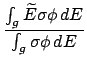and is given in the format,
     WRITE(iocmcf, '(1p, 6e11.4)')
& (e10barg(io), io = igsrt, igend)= 9:
This block of data is group-averaged photon multiplicity, number identifies the first of the list of secondary particles, and we have "multipl." for string. The flag line is followed by the same header as for= 3. The only difference is that the data identifier I_number is now 9. The data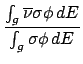is in the format,
     WRITE(iocmcf, '(1p, 6e11.4)')
& (array(j), j=igsrt, igend)= 10:
This block of data gives equiprobable angular bins. The number identifies the first of the list of secondary particles, and we have "ang(i=1)" for string. The flag line is followed by the header,
     WRITE(iocmcf, '(1p, 4i5, 5x, i5, 3e12.5)')
&  c, I_number, s, num_engy_in, neqpb, q0, x1

For this value of the flag the data identifier I_number is 1. The unusual item in the header is neqpb, the number of equiprobable angular bin edges. (The number of bins is one less.) The first data entries are the incident energies in the format,
     WRITE(iocmcf, '(1p, 6e11.4)')
& (energy_in(k), k=1, num_engy_in)

The equiprobable angular bin boundaries are concatenated into a single long array, with a block for each incident energy. The entries are printed in the format,
     WRITE(iocmcf, '(1p, 6e13.6)')
& (cpdi2(k), k=1, neqpb*num_engy_in)= 11:
This block of data gives correlated bins which are equally probable jointly with respect to angle and energy. The number in the flag line identifies the first of the list of secondary particles, and string is "ang(i=3)". The flag line is followed by the header,
     WRITE(iocmcf, '(1p, 6i5, 3e12.5)')
&  c, I_number, s, num_engy_in,
&  nppbe, neqint, q0, x1


Here, the value of I_number is 3. The number of equiprobable angular bin edges is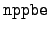, and the number of equiprobable energy bin edges is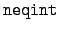. Just as for= 10 (equiprobable energy distributions), the block starts with the incident energies in the format,

     WRITE(iocmcf, '(1p, 6e11.4)')
& (energy_in(k), k=1, num_engy_in)


The joint energy-angle distributions are printed in sections. Each section contains, first, an equiprobable angular bin boundary point

     WRITE(iocmcf, '(1p, 6e11.4)')
& I1_equi_cos(k, engy_index)

There follows the equiprobable energy bin boundaries for this cosine,
     WRITE(iocmcf, '(1p, 6e13.6)')
& (equi_engy(j), j=1, neqint)= 12:
This flag has two possible meanings, and they ought to be split. In both cases number identifies the first of the list of secondary particles, and string is "e spec". The two meanings of the flag are distinguished by different header lines.= 12, Energy distributions:
If= 4 the flag 12 denotes equiprobable energy distributions. The header is,
    WRITE(iocmcf,'(1p, 4i5, 5x, i5, 3e12.5)')
& c, I_number, s, num_engy_in,
& neqpb, q0, x1

This is standard header information except for neqpb, the number of equiprobable energy bin edges. (The number of bins is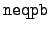- 1.) The data is in two sets. First, we have the incident energies,
    WRITE(iocmcf, '(1p, 6e11.4)')
& (energy_in(k), k=1 ,num_engy_in)

The equidistributed energy deposition bin boundaries for the various incident energies are concatenated into a single array, and this array is printed in the format,
    WRITE(iocmcf, '(1p, 6e13.6)')
& (cpdi2(k), k=1, neqpb*num_engy_in)= 12, Gamma production:
If= 1 and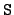= 3 (so that the data gives angular distribution for gamma production), this block appears before the angular distribution data (which has= 10). There is a special header,
    WRITE(iocmcf, '(1p, 5i5, 5x, 3e12.5)')
& c, I_number, s, 2, 2, q0, x1

In this case x1 is the energy of the ejected gamma. This line is followed by the energy range
    WRITE(iocmcf, '(1p, 6e11.4)') emin, emax

and by the energy of the ejected gamma, four more times!
    WRITE(iocmcf, '(1p, 4e13.6)') (x1, i0 = 1, 4)

Note that this is the only situation in which the values of emin and emax get printed, even though they are present with all of the data in the library' file.= 14:
This block of data gives coherent scattering form factors. In the flag line number identifies the first of the list of secondary particles, and string is "coh.scat". The flag line is followed by the header,
     WRITE(iocmcf, '(1p, 4i5, 2e12.5)')
& c, I_number, s, ndata, q0, x1

Here, the value of I_number is 941, and ndata is the length of the data. The scattering form factors are given in the format,
     WRITE(iocmcf, '(1p, 6e11.4)')
& (scats(j), j=1, ndata)= 15:
This block of data gives incoherent scattering form factors. In the flag line number identifies the first of the list of secondary particles, and string is "inc.scat". The header and the data block are the same as for= 14. The only difference is that I_number is 942.= 99:
There is no more data for this target.

Incidentally, if= 1 (grid-based data), there is an extra phoney-target line containing the interpolation type used for each value of I_number. The format of this line is,

     WRITE(iocmcf,'("999999" / "MCFGEN	  Date:", i8,
&     5x, "Library type:", i3,/,
&     3x, i3, " (0)  ",
&     i3, " (7)  ",
&     i3, " (9)  ",
&     i3, " (10) ",
&     i3, "(94x) ")'
&     ) i_date, 1, (interp_typ(count), count=1, 5)

These entries have the following meanings.

i_date:
The date the output file was made.

1:
Indicates grid-based data.

interp_typ, (I):
For the value I of I_number what sort of interpolation to use. The only values used are: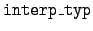= 0 :
Linear-linear interpolation.= 5 :Next: The format for mcf.bin' Up: The format for mcf.asc' Previous: The format for mcf.asc'   Contents